# Reptorian G'MIC Filters

Interesting - I have some doubts that would lead to heavy gains in practice actually. Agreed about variable naming of course, depends whether we expect anyone else to maintain it though…

Or just to be sociable. If one wants to converse in code `tis best to be transparently clear throughout. Say it three times: though variable name hinting, the code itself and telling comments.

If one just intends to be a hermit, name variables tersely to save keystrokes. Years on — or even months! — if once-clear code is now incomprehensible, there is but one person to blame and one to suffer.

In most places definitely - especially when working in teams. We sort of covered this before in another thread. Perhaps we should revive that or make another rather than in here, but in short: looking at the existing contributed code, I think variable naming is the least of our worries.

1 Like

I kind of agree with @garagecoder.
There is a current trend that code must be readable (and well documented!) to be worthy of consideration. And this simplistic idea has made its way in the software development world.
Although this is indeed a desirable property in many cases (team development and so on…), the readability of a code is ultimately only a very minor aspect of the “value” of a program.

For example, if I want to do something quick, only for me, knowing that I won’t go back to it in 2 years or even 2 weeks, and I use a clever way of solving my problem. That’s not bad code, even if the variables I use are named `a`, `b` and `c`.
That would be clearly better than having a very well documented code with fancy variable names that takes forever to solve the same problem, because the algorithm used there just sucks.

Evaluating the quality of a code under the only aspect of its readability is in my opinion a serious mistake.

Would it mean that all programs participating in code obfuscation contests would be considered “bad”? This is exactly the contrary : those are treasures of ingenuity and creativity that only a few talented programmers are able to achieve.

Don’t get me wrong : I don’t say code readability is a bad practice.
It’s just not the only measure to consider chen evaluating the quality of a code.

I think that developers too much focused on the readability of the code should do a small internship of 2 or 3 months doing assembly programming, it would open their mind1 Like

@grosgood I just have to say that one place where code clarity is very much appreciated (and indeed required) is of course your tutorials!

Not everything must be in extremes; g’mic code is capable of being readable, when we need it to be. I do try and keep some sort of balance in general.

So as not to further hijack @Reptorian’s thread, I’ve placed my thoughts over at Tutorial Fragments.

1 Like

I changed Default Input Mode, and I’m not sure if I did it right. Let me know if it is wrong.

It doesn’t work in Krita, but I filed a report for that.

How to get around this?

``````Function 'da_remove()': Invalid starting (0) and ending (0) positions (not ordered, in range -0...-1).
``````

My workaround shows that I have failed unfortunately at a more easier to understand rep_dla, ah well, I think I will make a new version of rep_dla. I discovered a new technique involving a image representing a string, and using replace_str to do similar thing to what I did in there.

I think that’s happening because you dynamic array contains 0 elements.
PS this error occurs in this simple case:

``````\$ gmic 1,2 eval "da_remove(0)"
``````

I think I’m gonna improve the error message in that case though.

EDIT: Done.

Will be available for the next release.

1 Like

Thanks, I had to do `if(da_size(#A),da_remove(#A,n););` in the code of refactored rep_dla. Other than that, the output are the same or similar

That being said, hmm, I’ll have to figure out how to improve it now that it’s far more readable as I have removed global variable, and removed the `dar_lines.=` blocks.

I may actually have a faster version of rep_dla soon. I figured some things out.Saving this code for later to finish up on optimized **rep_dla**
``````length_const_line="begin_t(
const ww=w#0;
const hh=h#0;
const lim_atmp="\$2";
const dl_mode="{(\$4%2)>0?1:0}";
const use_border=\$check_count;"

length_const_line.="
newpos_x=[-1,0,1,-1,1,-1,0,1];
newpos_y=[-1,-1,-1,0,0,1,1,1];
);
attempts=0;"

if \$3==3 length_const_line.=altern=round(u); fi

('"
t==N?(
do(n=0;
do(
temp_vec=I[#A,n];
xp=temp_vec;
yp=temp_vec;
if(C,
i(#0,xp%ww,yp%hh)=dl_mode;
if(da_size(#A),da_remove(#A,n););
--n;
attempts=0;
);
++n;
,n<da_size(#A)
);
repeat(da_size(#A),p,
temp_vec=I[#A,p];
px=temp_vec;
py=temp_vec;
pv=int(u(0,8));
npx=newpos_x[pv];
npy=newpos_y[pv];
I[#A,p]=[px+npx,py+npy];
);
++attempts;\n
D
,da_size(#A)&&(attempts<lim_atmp)
);
)
"')

if \$3==3
replace_str. "D","altern=++altern%2;"
else
replace_str. "D",""
fi

if \$3==0 C_replace_str="
i(#0,xp-1,yp-1,0,0,0,2)<1||
i(#0,xp-1,yp+1,0,0,0,2)<1||
i(#0,xp+1,yp-1,0,0,0,2)<1||
i(#0,xp+1,yp+1,0,0,0,2)<1"
elif \$3==1 C_replace_str="
i(#0,xp-1,yp,0,0,0,2)<1||
i(#0,xp+1,yp,0,0,0,2)<1||
i(#0,xp,yp-1,0,0,0,2)<1||
i(#0,xp,yp+1,0,0,0,2)<1"
elif \$3==2 C_replace_str="
i(#0,xp-1,yp-1,0,0,0,2)<1||
i(#0,xp-1,yp+1,0,0,0,2)<1||
i(#0,xp+1,yp-1,0,0,0,2)<1||
i(#0,xp+1,yp+1,0,0,0,2)<1||
i(#0,xp-1,yp,0,0,0,2)<1||
i(#0,xp+1,yp,0,0,0,2)<1||
i(#0,xp,yp-1,0,0,0,2)<1||
i(#0,xp,yp+1,0,0,0,2)<1"
elif \$3==3 C_replace_str="
altern?(
i(#0,xp-1,yp-1,0,0,0,2)<1||
i(#0,xp-1,yp+1,0,0,0,2)<1||
i(#0,xp+1,yp-1,0,0,0,2)<1||
i(#0,xp+1,yp+1,0,0,0,2)<1
):(
i(#0,xp-1,yp,0,0,0,2)<1||
i(#0,xp+1,yp,0,0,0,2)<1||
i(#0,xp,yp-1,0,0,0,2)<1||
i(#0,xp,yp+1,0,0,0,2)<1
)"
fi

if \$4%2
(\$C_replace_str)
replace_str. "<1",""
C_replace_str={t}
rm.
fi

replace_str. "C",\$C_replace_str

store. dar_lines_string

insert_dar_pos={\$>+1}

\$dar_lines_string

replace_str. "N",\$>
replace_str. "A",\$insert_dar_pos

dar_lines.={t}

if (\$>!=\$mt)
dar_lines.=:
else
dar_lines.=;
fi

rm.

}
``````

Looks like I failed in finishing my huge refactor and improvement. But, I’d appreciate last minute code review. I’ll do some code review in a new thread.

Imma save my code here. Not ready for production use. Easier to read, faster too.

Saving rep_dla refactored code here
``````#@cli rep_dla : eq. to 'rep_diffusion_limited_aggregation' : (+)
rep_dla_new: rep_diffusion_limited_aggregation_new \$*
#@cli : Generate Diffusion Limited Aggregation
#@cli : (eq. to 'rep_dla').\n
#@cli : '_point_proximity' defines how frequently noises are generated based on the proximity of pixels. The lower the number, the higher the density.
#@cli : '_escape' defines how much attempts on filling the aggregation form before finishing.
#@cli : '_mode' defines how particles aggregate on the aggregation form.
#@cli : '_target' defines where the aggregation form will fill on.
#@cli : '_border' is only applicable if there is a planting seed map. It is used to limit particles based on proximity away from existing structure.
#@cli : '_preserve_binary_image' is only applicable on images with variance.
#@cli : Author: Reptorian.
rep_diffusion_limited_aggregation_new:
skip \${1=2},\${2=100},\${3=1},\${4=0},\${5=0},\${6=1}

bg,use_altern_text={\$4?1:0},{(\$3%4)==3}

m "dla_target_2s : n 0,1 s. c * midpoint={avg(iM,im)} f. i>\$midpoint?1"
m "dla_target_3s : n 0,1 s c add / 3 midpoint={avg(iM,im)} f. i>\$midpoint?1"
m "dla_target_4s_plus : n 0,1 ts={s-1} s c add[^-1] /.. \$ts * midpoint={avg(iM,im)} f. i>\$midpoint?1"
m "dla_target : if s==1 n 0,1 elif s==2 dla_target_2s elif s==3 dla_target_3s elif s>3 dla_target_4s_plus fi"
m "dla_check_variance : tv=0 repeat s sh \$> tv+={iv#-1} rm. if \$tv break fi done u {\$tv?1:0}"
m "dla_clear_image : {w#0},{h#0},1,1,"{\$4?0:1}" rv[-1,0] rm."

if \$use_altern_text
altern_text_a=altern=int(u(0,2))
altern_text_b=altern=++altern%2
fi

foreach {

if s>4||d#0>1 continue fi

+dla_create_coordinate_map \$1
gcd_shuffle.

use_dla_map=\${dla_check_variance}

if \$use_dla_map

dla_target

if !\$6
if \$4
* .001
else
replace 0,.999
fi
fi

if \$border
if \$4 +dilate_circ \$border
else  +erode_circ \$border
fi

1,{h},1,1,y
eval[-3] :if(i(#0,I)==i(#-2,I),i(#-1,0,y)=-1);I;
map[-1] [-3]
rv[-3,-1]
rm[-2,-1]

else
if \$4 val_check=>.999
else  val_check=<.001
fi

1,{h},1,1,y
eval.. :if(!(i(#0,I)\$val_check),i(#-1,0,y)=-1;);I;
map. ..
rm..

+distance[-2] {!\$4},2
1,{h#-2},1,1,i(#-1,I(#-2))
rm[-2]
pixelsort[-2] +,y,[-1]
rm[-1]
map. ..
rm..

fi

else

center_x,center_y={[(w#0-1)>>1,(h#0-1)>>1]}

1,{h},1,1,norm(I#-1-[\$center_x,\$center_y])
pixelsort.. +,y,.
rm.

dla_clear_image
set \$bg,50%,50%

fi

a[^0] y

eval :"begin_t(
const width=w#0;
const height=h#0;
const limit_of_attempts=\$2;
const pixel_detection_mode=\$3%4;
const target_mode=!(\$4&1);
new_pos_x=[-1, 0, 1,
-1,    1,
-1, 0, 1];
new_pos_y=[-1,-1,-1,
0,    0,
1, 1, 1];
!target_mode?(
pixel_detection_mode==3?(
pixel_detected()=
altern?(
i(#0,xp-1,yp-1,0,0,0,2)<1||
i(#0,xp-1,yp+1,0,0,0,2)<1||
i(#0,xp+1,yp-1,0,0,0,2)<1||
i(#0,xp+1,yp+1,0,0,0,2)<1
):(
i(#0,xp-1,yp,0,0,0,2)<1||
i(#0,xp+1,yp,0,0,0,2)<1||
i(#0,xp,yp-1,0,0,0,2)<1||
i(#0,xp,yp+1,0,0,0,2)<1
);
):
pixel_detection_mode==2?(
pixel_detected()=
i(#0,xp-1,yp-1,0,0,0,2)<1||
i(#0,xp-1,yp+1,0,0,0,2)<1||
i(#0,xp+1,yp-1,0,0,0,2)<1||
i(#0,xp+1,yp+1,0,0,0,2)<1||
i(#0,xp-1,yp,0,0,0,2)<1||
i(#0,xp+1,yp,0,0,0,2)<1||
i(#0,xp,yp-1,0,0,0,2)<1||
i(#0,xp,yp+1,0,0,0,2)<1;
):
pixel_detection_mode==1?(
pixel_detected()=
i(#0,xp-1,yp,0,0,0,2)<1||
i(#0,xp+1,yp,0,0,0,2)<1||
i(#0,xp,yp-1,0,0,0,2)<1||
i(#0,xp,yp+1,0,0,0,2)<1;
):(
pixel_detected()=
i(#0,xp-1,yp-1,0,0,0,2)<1||
i(#0,xp-1,yp+1,0,0,0,2)<1||
i(#0,xp+1,yp-1,0,0,0,2)<1||
i(#0,xp+1,yp+1,0,0,0,2)<1;
);
):(
pixel_detection_mode==3?(
pixel_detected()=
altern?(
i(#0,xp-1,yp-1,0,0,0,2)||
i(#0,xp-1,yp+1,0,0,0,2)||
i(#0,xp+1,yp-1,0,0,0,2)||
i(#0,xp+1,yp+1,0,0,0,2)
):(
i(#0,xp-1,yp,0,0,0,2)||
i(#0,xp+1,yp,0,0,0,2)||
i(#0,xp,yp-1,0,0,0,2)||
i(#0,xp,yp+1,0,0,0,2)
);
):
pixel_detection_mode==2?(
pixel_detected()=
i(#0,xp-1,yp-1,0,0,0,2)||
i(#0,xp-1,yp+1,0,0,0,2)||
i(#0,xp+1,yp-1,0,0,0,2)||
i(#0,xp+1,yp+1,0,0,0,2)||
i(#0,xp-1,yp,0,0,0,2)||
i(#0,xp+1,yp,0,0,0,2)||
i(#0,xp,yp-1,0,0,0,2)||
i(#0,xp,yp+1,0,0,0,2);
):
pixel_detection_mode==1?(
pixel_detected()=
i(#0,xp-1,yp,0,0,0,2)||
i(#0,xp+1,yp,0,0,0,2)||
i(#0,xp,yp-1,0,0,0,2)||
i(#0,xp,yp+1,0,0,0,2);
):(
pixel_detected()=
i(#0,xp-1,yp-1,0,0,0,2)||
i(#0,xp-1,yp+1,0,0,0,2)||
i(#0,xp+1,yp-1,0,0,0,2)||
i(#0,xp+1,yp+1,0,0,0,2);
);
);
"\$altern_text_a"
);
temp_vec=I;
xp=temp_vec;
yp=temp_vec;
repeat(limit_of_attempts,attempts,
if(pixel_detected(),
i(#0,xp,yp)=target_mode;
break();
);
pv=int(u(0,8));
xp+=new_pos_x[pv];
yp+=new_pos_y[pv];
xp%=width;
yp%=height;
"\$altern_text_b"
);
[0,0];
"

k

if \$use_dla_map&&!\$6 round fi

}

um dla_target,dla_target_2s,dla_target_3s,dla_target_4s_plus,dla_create_coordinate_map
+dla_create_coordinate_map:
if \$1
row_a={ceil(h/2)}
row_b={h-\$row_a}
counts_per_row_a,counts_per_row_b={[w,w+1]>>1}

1,{\$row_a*\$counts_per_row_a+\$row_b*\$counts_per_row_b},1,2,:"begin(
const row_length=w#-1;
const half_row_length=row_length>>1;
);
pos_y=int(y/half_row_length);
ny=y<<1;
pos_x=pos_y&1?(ny%row_length):((ny+1)%row_length);
[pos_x,pos_y];
"
else
1,{(w>>1)*(ceil(h/2))},1,2,:"begin(
const row_length=w#-1;
const half_row_length=row_length>>1;
);
y_pos=int(y/half_row_length)<<1;
[(y<<1)%row_length,y_pos];
"
fi
``````

Also, thank you @garagecoder for making gcd_shuffle. This allows me to rearrange coordinate map pixels for more randomization.

Picture of refactored rep_dla result.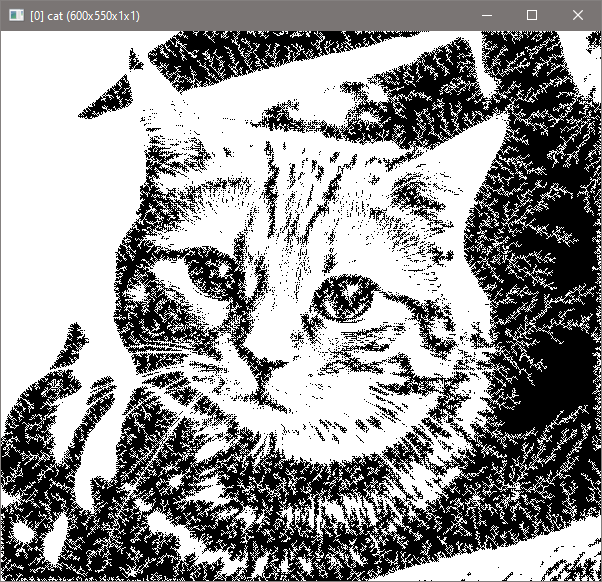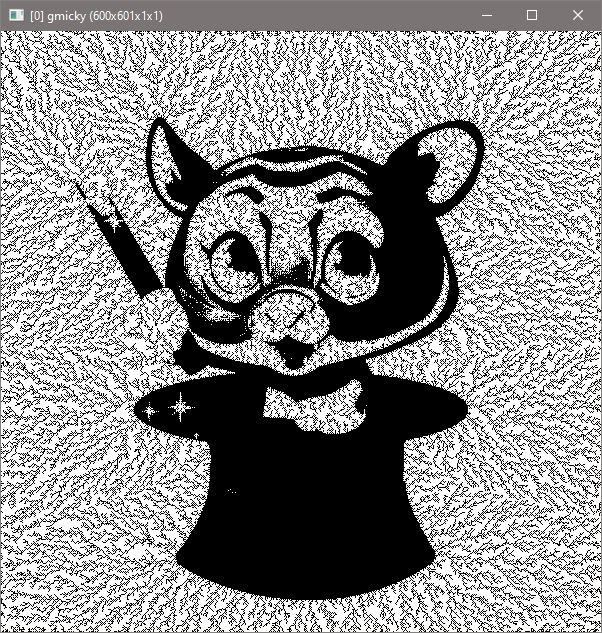Hmm, it’s interesting how this flows along edge. I never made that feature in mind. Seem like it’s the result of distance-based sorting.

Also, I found that the new version is more beautiful. Here’s the old version result with identical setting.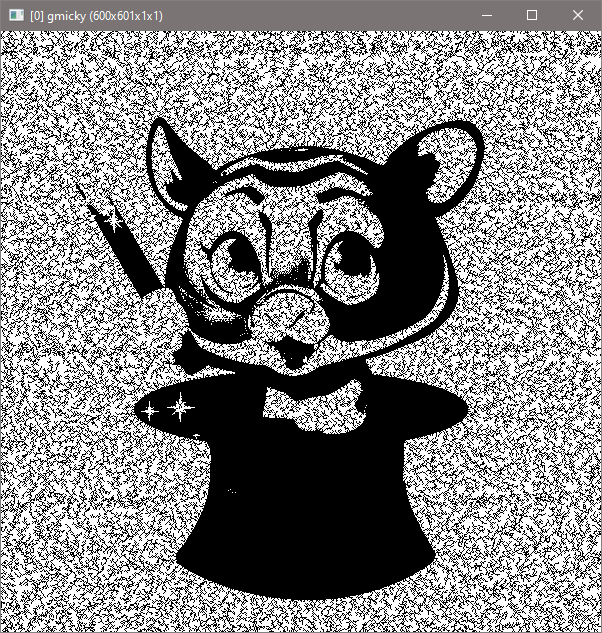Here’s the performance too:

``````C:\Windows\System32>gmic sp gmicky tic rep_dla_new 0,500,2,1 toc
[gmic]-0./ Start G'MIC interpreter.
[gmic]-1./ Input sample image 'gmicky' (1 image 600x601x1x3).
[gmic]-1./ Initialize timer.
[gmic]-1./ Elapsed time: 0.247 s.
[gmic]-1./ Display image  = 'gmicky'.
 = 'gmicky':
size = (600,601,1,1) [1408 Kio of float32].
data = (1,1,1,0,0,0,1,1,0,0,1,1,(...),0,1,1,1,0,0,0,1,1,1,1,1).
min = 0, max = 1, mean = 0.579953, std = 0.493567, coords_min = (3,0,0,0), coords_max = (0,0,0,0).
[gmic]-1./ End G'MIC interpreter.

C:\Windows\System32>gmic sp gmicky tic rep_dla 0,500,2,0 toc
[gmic]-0./ Start G'MIC interpreter.
[gmic]-1./ Input sample image 'gmicky' (1 image 600x601x1x3).
[gmic]-1./ Initialize timer.
[gmic]-1./ Elapsed time: 1.497 s.
[gmic]-1./ Display image  = 'gmicky'.
 = 'gmicky':
size = (600,601,1,1) [1408 Kio of float32].
data = (1,1,1,0,1,0,1,1,1,1,1,1,(...),1,1,1,1,1,1,1,1,1,1,1,1).
min = 0, max = 1, mean = 0.53822, std = 0.498538, coords_min = (3,0,0,0), coords_max = (0,0,0,0).
[gmic]-1./ End G'MIC interpreter.
``````
2 Likes

Have a look at this sweet new code for rep_diffusion_limited_aggregation. Note there is a ‘_new’ next to command name, so it won’t interfere with current version. This will replace current version when I add a feature to randomly insert points via probability around appended points, and this will create thickening.

I say the code is sweet because it is easier to understand what’s going on and easier to add features or base new code off from. It’s faster too for all cases.

Also, I note that there is Laplacian Growth version, but I never was able to find code for it. Laplacian Growth is a much faster version for DLA.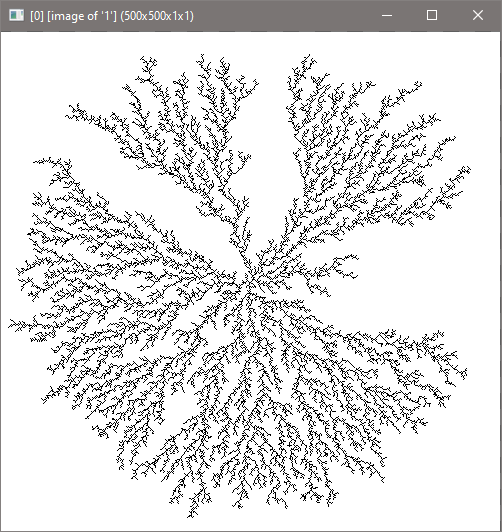The New Diffusion Limited Aggregation Code
``````\$ {vector2(300)}  rep_diffusion_limited_aggregation_new 100,2,0,0,0,0
#@cli rep_dla : eq. to 'rep_diffusion_limited_aggregation' : (+)
rep_dla_new: rep_diffusion_limited_aggregation_new \$*
#@cli rep_diffusion_limited_aggregation: _escape,_mode,_stem_color={ 0=dark | 1=light },_border,_initial_point_mode={ 0=less_dense | 1=more_dense },_preserve_binary_image={ 0=maskless | 1=mask }
#@cli : Generate Diffusion Limited Aggregation
#@cli : (eq. to 'rep_dla').
#@cli :
#@cli : '_escape' defines how much attempts on filling the aggregation form before finishing.
#@cli : '_mode' defines how particles aggregate on the aggregation form.
#@cli : '_stem_color' defines where the aggregation form will fill on.
#@cli : '_border' is only applicable if there is a planting seed map. It is used to limit particles based on proximity away from existing structure.
#@cli : '_initial_point_mode' defines whether to use less dense or more dense point coordinates map.
#@cli : '_preserve_binary_image' is only applicable on images with variance.
#@cli :
#@cli : Author: Reptorian.
rep_diffusion_limited_aggregation_new:
skip \${1=100},\${2=0},\${3=0},\${4=0},\${5=1},\${6=1}

maximum_iterations,pixel_detection_mode,stem_color,border_size,initial_coordinates_map_mode,preserve_silhouette_mode={[abs(\$1),int(\$2)%4,!\$3,round(abs(\$4)),\$5?1,\$6?1]}

m "dla_target_1s:
ge {avg(iM,im)}
"
m "dla_target_2s:
normalize 0,1
split[-1] c *
ge {avg(iM,im)}
"
m "dla_target_3s:
normalize 0,1
split c
ge {avg(iM,im)}
"
m "dla_target_4s:
normalize 0,1
ts={s-1}
split c
/[-1] \$ts *
ge {avg(iM,im)}
"
m "dla_target:
if s==1   dla_target_1s
elif s==2 dla_target_2s
elif s==3 dla_target_3s
else      dla_target_4s
fi
"
m "dla_check_variance:
tv=0

repeat s {
shared \$>
tv+={iv#-1}
rm.

if \$tv
break
fi
}

status {\$tv?1:0}
"
m "dla_clear_image:
{w#0},{h#0},1,1,"\$stem_color"
rv[-1,0]
rm.
"

if \$use_altern_text
altern_text_a=altern=int(u(0,2))
altern_text_b=altern=++altern%2
fi

foreach

if s>4||d#0>1 continue fi

+dla_create_coordinate_map \$initial_coordinates_map_mode
gcd_shuffle.

use_dla_map=\${dla_check_variance}

if \$use_dla_map

dla_target

if \$border_size
+distance.. \$inv_stem_color,2
1,{h#-2},1,1,v=i(#-1,I(#-2));v?(v<=\$border_size?y:-1):-1
map. ...
1,100%,1,1,i(#2,I(#-1))
rm[-4,-3]
pixelsort.. +,y,.
rm.
map. ..
rm..

if !\$preserve_silhouette_mode
if \$stem_color
replace 0,.999
else
* .001
fi
fi

else
if \$stem_color val_check=>.999
else  val_check=<.001
fi

1,{h},1,1,y
eval.. :if(!(i(#0,I)\$val_check),i(#-1,0,y)=-1;);I;
map. ..
rm..

+distance[-2] \$inv_stem_color,2
1,{h#-2},1,1,i(#-1,I(#-2))
rm[-2]
pixelsort[-2] +,y,[-1]
rm[-1]
map. ..
rm..

if !\$preserve_silhouette_mode
if \$stem_color
replace 0,.999
else
* .001
fi
fi

fi

else

center_x,center_y={[(w#0-1)>>1,(h#0-1)>>1]}

1,{h},1,2,"begin(
const sqrt_of_two=sqrt(2);
const center_x=\$center_x;
const center_y=\$center_y;
);
distance_from_center=norm(I#-1-[\$center_x,\$center_y]);
valid_point=lerp(1,u^.625,relative_position)>=relative_position?y:-1;
):(
valid_point=-1;
);
[distance_from_center,valid_point];
"

split[-1] c
[-1]
repeat 2 { map[{\$>-2}] [{\$>-4}] }
remove[-4,-3]
pixelsort[-2] +,y,[-1]
remove[-1]
map[-1] [-2]
remove[-2]

dla_clear_image
set \$inv_stem_color,50%,50%
fi

a[^0] y

eval :"begin_t(
const width=w#0;
const height=h#0;

const limit_of_attempts=\$maximum_iterations;
const pixel_detection_mode=\$pixel_detection_mode;
const target_mode=\$inv_stem_color;

new_pos_x=[-1, 0, 1,
-1,    1,
-1, 0, 1];
new_pos_y=[-1,-1,-1,
0,    0,
1, 1, 1];

if(!target_mode
,det_px(pixel)=pixel<1;
,det_px(pixel)=pixel;
);

pixel_detection_mode==3?(
pixel_detected()=
altern?(
det_px(i(#0,xp-1,yp-1,0,0,0,2))||
det_px(i(#0,xp-1,yp+1,0,0,0,2))||
det_px(i(#0,xp+1,yp-1,0,0,0,2))||
det_px(i(#0,xp+1,yp+1,0,0,0,2))
):(
det_px(i(#0,xp-1,yp,0,0,0,2))||
det_px(i(#0,xp+1,yp,0,0,0,2))||
det_px(i(#0,xp,yp-1,0,0,0,2))||
det_px(i(#0,xp,yp+1,0,0,0,2))
);
):
pixel_detection_mode==2?(
pixel_detected()=
det_px(i(#0,xp-1,yp-1,0,0,0,2))||
det_px(i(#0,xp-1,yp+1,0,0,0,2))||
det_px(i(#0,xp+1,yp-1,0,0,0,2))||
det_px(i(#0,xp+1,yp+1,0,0,0,2))||
det_px(i(#0,xp-1,yp,0,0,0,2))||
det_px(i(#0,xp+1,yp,0,0,0,2))||
det_px(i(#0,xp,yp-1,0,0,0,2))||
det_px(i(#0,xp,yp+1,0,0,0,2));
):
pixel_detection_mode==1?(
pixel_detected()=
det_px(i(#0,xp-1,yp,0,0,0,2))||
det_px(i(#0,xp+1,yp,0,0,0,2))||
det_px(i(#0,xp,yp-1,0,0,0,2))||
det_px(i(#0,xp,yp+1,0,0,0,2));
):(
pixel_detected()=
det_px(i(#0,xp-1,yp-1,0,0,0,2))||
det_px(i(#0,xp-1,yp+1,0,0,0,2))||
det_px(i(#0,xp+1,yp-1,0,0,0,2))||
det_px(i(#0,xp+1,yp+1,0,0,0,2));
);

"\$altern_text_a"
);

temp_vec=I;

xp=temp_vec;
yp=temp_vec;

repeat(limit_of_attempts,
pv=int(u(0,8));

xp+=new_pos_x[pv];
yp+=new_pos_y[pv];
xp%=width;
yp%=height;

if(pixel_detected(),
i(#0,xp,yp)=target_mode;
break();
);

"\$altern_text_b"
);

I;
"

k

if \$use_dla_map&&!\$preserve_silhouette_mode round fi

done

um dla_target,dla_target_1s,dla_target_2s,dla_target_3s,dla_target_4s,dla_check_variance,dla_clear_image
+dla_create_coordinate_map:
if \$1
row_a={ceil(h/2)}
row_b={h-\$row_a}
counts_per_row_a,counts_per_row_b={[w,w+1]>>1}

1,{\$row_a*\$counts_per_row_a+\$row_b*\$counts_per_row_b},1,2,:"begin(
const row_length=w#-1;
const half_row_length=row_length>>1;
);
pos_y=int(y/half_row_length);
ny=y<<1;
pos_x=pos_y&1?(ny%row_length):((ny+1)%row_length);
[pos_x,pos_y];
"
else
1,{(w>>1)*(ceil(h/2))},1,2,:"begin(
const row_length=w#-1;
const half_row_length=row_length>>1;
);
y_pos=int(y/half_row_length)<<1;
[(y<<1)%row_length,y_pos];
"
fi
``````

EDIT: I realized my algorithm fails in one way. It seems that there isn’t as much perpendicular stem at all, and that is needed in case of empty image. Hmm, that needs to be addressed. I don’t know, but smaller images doesn’t have that problem: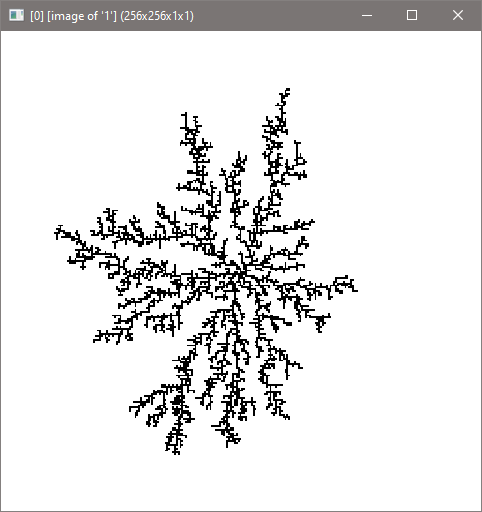I have added a new feature to Diffusion Limited Aggregation which is spread_factor. Basically, it thickens the stems.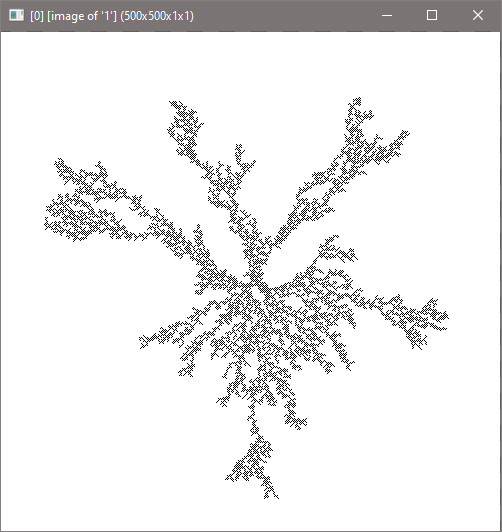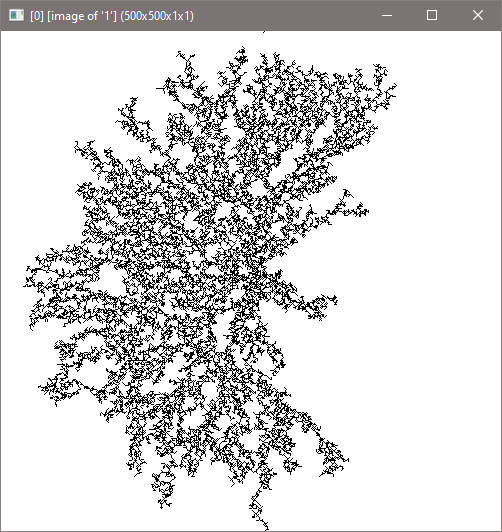Not sure how to exactly implement it, but I’ll take it.

EDIT: I pushed the upgrade to Diffusion Limited Aggregation.

Ok, I fixed spread factor, now it works properly: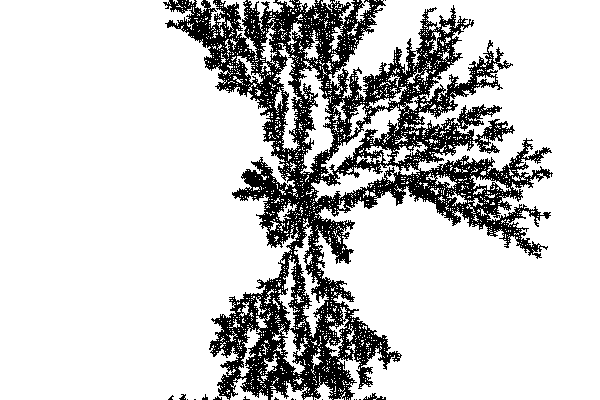Another example: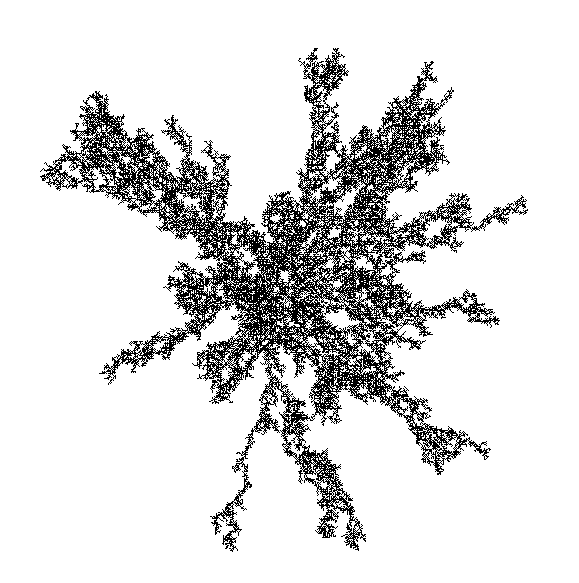Looks like I have solved the pal problem. I am able to refactor it and remove 300+ subcommands. Speed is almost kept too. A tiny bit little slower, but not by far. Only noticeable via timer. Reasonable when considering multiple subcommands can slow everything else down. Never mind. Close though. I’ll just be keeping the current solution with a few update. Faster now at least.

Guess what I managed to create in G’MIC without the use of BIGINT library?

Still, some bugs though. I’m not sure how to properly convert a image representing a large number into a image of binary yet it seems. Ooh, so close. I wish dec2bin and bin2dec would allow for humongous digits for this case or something like that.

``````\$ 106,17 rep_tupper
#@cli rep_tupper: k
#@cli : Generates output of K onto images.
rep_tupper:
skip \${1=960939379918958884971672962127852754715004339660129306651505519271702802395266424689642842174350718121267153782770623355993237280874144307891325963941337723487857735749823926629715517173716995165232890538221612403238855866184013235585136048828693337902491454229288667081096184496091705183454067827731551705405381627380967602565625016981482083418783163849115590225610003652351370343874461848378737238198224849863465033159410054974700593138339226497249461751545728366702369745461014655997933798537483143786841806593422227898388722980000748404719}
check "\$#==1"
fill "y+x*h" mirror. x

decimal_number=\$1

1 # 1. Insert Binary Here

1 # 2. Create single pixel image for use dynamic processing.

eval "
number='\$1';
const divisor=h#0;
const n_size=size(number);
const n_0=_'0';
index=0;
dividend=number[index]-n_0;

while(dividend<divisor,
dividend=dividend*10+(number[++index]-n_0);
);

while(n_size>index,
da_push(#-1,int(dividend/divisor)+n_0);
dividend=(dividend%divisor)*10+(number[++index]-n_0);
);

da_push(#-2,(i[#-1,da_size(#-1)-1]-n_0)&1);
ds=da_size(#-1);
ds?da_freeze(#-1);
"

decimal_number={t}

repeat inf {
rm. 1

eval "
number='"\$decimal_number"';
const divisor=2;
const n_size=size(number);
const n_0=_'0';
index=0;
dividend=number[index]-n_0;

while(dividend<divisor,
dividend=dividend*10+(number[++index]-n_0);
);

while(n_size>index,
da_push(#-1,int(dividend/divisor)+n_0);
dividend=(dividend%divisor)*10+(number[++index]-n_0);
);

da_push(#-2,(i[#-1,da_size(#-1)-1]-n_0)&1);
ds=da_size(#-1);
ds?da_freeze(#-1);
"

decimal_number={t}

if h#-1==1 break fi
}

rm. eval da_freeze(#-1)

map.. . rm.
``````

EDIT: I fixed the bug.It looks like this fun filter is happening though I can’t imagine a real use case of this other than teaching students how loading and exporting file work.

Any one have a way of converting a image of binary number to a image that represents decimal number? All commands I know of so far can’t utilize huge binary number akin to ones that don’t fit in doubles.

For example, this:

``````1100000000000000011111011000000000011111110000000000000111100000000000000011000000000000000000001000000000000000110000000000000000000110000110000000001111101100000000001111111000000000000011100001111000000001000111110000000000001100101110000000011100111111000000011111001111000000011110000110000000000111101100000000000111110001000000000011000011110011000000000011111111110000000001111111110000000000010001100000000000000001000011100000011110001111110000111000010001110000000000110001100000000001111111000100000000111100011100000000000000111000111100000001110011111110000001000110001100000000001110001000000000001111110000000000000111000011100000000000000111000000000000000000011100110000000000111111100000000000001111000111100000000010011111100000000000110001011110000000111100111110000000111111000110000000000111000100011110000111111001111111000000000011000110000000000011110100000000000011111011001000000000000111110001111000000011100011111100000000000100001000000000001111110001110000001111100011110000000000000001100011110000001111001111110000011100011000110000000000011111100110000000011110001001000000000000011111000000000000001110011000100000000000111111000000000000000110001100000000000000011110000000000000000110011111000000001100100011000011010001110010001111000001111100010000000000000000111100000110000000111100111111000000001001000010001111100011111100010011000011111000110000000000000001111100000000000000000000000010000000000011111110000000100000111000111111000000000000000110000000000000000000111110000000000001100110001111100011000100110001100111111001111111000000000000111100000000000000000000000010000111100011111110011111100011111100110001000000000000111110000000000000000000000000000000000000000000000000000
``````

Using bitwise shifting?